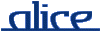# alice library manual.# The VectorPair structure

## ________ Synopsis ____________________________________________________

```    signature VECTOR_PAIR
structure VectorPair : VECTOR_PAIR
```

The VectorPair structure provides operations on pairs of vectors, very much like the ListPair structure does for pairs of lists. These operations do not require that the vectors have the same length; when they are of uneven lengths, the excess elements from the longer vector are ignored.

See also: Vector, ListPair

## ________ Import ______________________________________________________

Imported implicitly.

## ________ Interface ___________________________________________________

```    signature VECTOR_PAIR =
sig
val zip :     'a vector * 'b vector -> ('a * 'b) vector
val unzip :   ('a * 'b) vector -> 'a vector * 'b vector

val app :     ('a * 'b -> unit) -> 'a vector * 'b vector -> unit
val appr :    ('a * 'b -> unit) -> 'a vector * 'b vector -> unit
val map :     ('a * 'b -> 'c) -> 'a vector * 'b vector -> 'c vector
val foldl :   ('a * 'b * 'c ->'c) -> 'c -> 'a vector * 'b vector -> 'c
val foldr :   ('a * 'b * 'c ->'c) -> 'c -> 'a vector * 'b vector -> 'c
val all :     ('a * 'b -> bool) -> 'a vector * 'b vector -> bool
val exists :  ('a * 'b -> bool) -> 'a vector * 'b vector -> bool
val find :    ('a * 'b -> bool) -> 'a vector * 'b vector -> ('a * 'b) option

val appi :    (int * 'a * 'b -> unit) -> 'a vector * 'b vector -> unit
val appri :   (int * 'a * 'b -> unit) -> 'a vector * 'b vector -> unit
val mapi :    (int * 'a * 'b -> 'c) -> 'a vector * 'b vector -> 'c vector
val foldli :  (int * 'a * 'b * 'c -> 'c) -> 'c -> 'a vector * 'b vector -> 'c
val foldri :  (int * 'a * 'b * 'c -> 'c) -> 'c -> 'a vector * 'b vector -> 'c
val alli :    (int * 'a * 'b -> bool) -> 'a vector * 'b vector -> bool
val existsi : (int * 'a * 'b -> bool) -> 'a vector * 'b vector -> bool
val findi :   (int * 'a * 'b -> bool) -> 'a vector * 'b vector -> (int * 'a * 'b) option
end
```

## ________ Description _________________________________________________

zip (vec1, vec2)

Combines the two vectors vec1 and vec2 into a vector of pairs, with the first element of each vector comprising the first element of the result, the second elements comprising the second element of the result, and so on. If the vectors are of unequal lengths, the excess elements from the longer one are ignored.

unzip vec

Returns a pair of vectors formed by splitting the elements of vec. This is the inverse of zip for equal length vectors.

map f (vec1, vec2)

Maps the function f over the vector of pairs of elements from from the vectors vec1 and vec2, returning the vector of results. If the vectors are of unequal lengths, the excess elements from the longer one are ignored. The following equivalence holds:

`        map f (vec1, vec2) = Vector.map f (zip (vec1, vec2))`
app f (vec1, vec2)
appr f (vec1, vec2)

Applies the function f to the vector of pairs of elements from from the vectors vec1 and vec2 in left to right order (right to left, respectively). If the vectors are of unequal lengths, the excess elements from the longer one are ignored. The following equivalences hold:

```        app  f (vec1, vec2) = Vector.app  f (zip (vec1, vec2))
appr f (vec1, vec2) = Vector.appr f (zip (vec1, vec2))```
foldl f b (vec1, vec2)
foldr f b (vec1, vec2)

Return the result of folding the function f over the pair of vectors vec1 and vec2. The following equivalences hold:

```        foldl f b (vec1, vec2) = Vector.foldl f' b (zip (vec1, vec2))
foldr f b (vec1, vec2) = Vector.foldr f' b (zip (vec1, vec2))```

where f' is fn ((a,b),c) => f(a,b,c).

all f (vec1, vec2)
exists f (vec1, vec2)

These functions provide short-circuit testing of a predicate f over a pair of vectors. The following respective equivalences hold:

```         all f (vec1, vec2) = Vector.all f (zip (vec1, vec2))
exists f (vec1, vec2) = Vector.exists f (zip (vec1, vec2))```
find f (l1, l2)

Applies f to each pair (x1,x2) of elements of the vectors, from left to right, until f(x1,x2) evaluates to true; returns SOME(x1,x2) if such a pair exists and NONE otherwise. If the vectors are of unequal lengths, the excess elements of the longer one are ignored. The above expression is equivalent to:

`         Vector.find f (zip (vec1, vec2))`
appi f (l1, l2)
appri f (l1, l2)
mapi f (l1, l2)
foldli f b (l1, l2)
foldri f b (l1, l2)
alli f (l1, l2)
existsi f (l1, l2)
findi f (l1, l2)

Indexed versions of the functions app, appr, map, foldl, foldr, all, exists and findi. The index of each element is passed to f as an additional argument. For appri and foldri, processing starts at the highest index. In the case of success, findi returns the index of the pair of elements found along with the respective elements. The following equivalences hold:

```        app f (l1, l2)     = appi (f o g1) (l1, l2)
appr f (l1, l2)    = appri (f o g1) (l1, l2)
map f (l1, l2)     = mapi (f o g1) (l1, l2)
foldl f b (l1, l2) = foldli (f o g2) b (l1, l2)
foldr f b (l1, l2) = foldri (f o g2) b (l1, l2)```
all f (l1, l2) = alli (f o g1) (l1, l2) exists f (l1, l2) = existsi (f o g1) (l1, l2) find f (l1, l2) = Option.map g1 (foldri (f o g1) (l1, l2))

where

```        g1 = fn (i,a,b) => f(a,b)
g2 = fn (i,a,b,c) => f(a,b,c)    ```

last modified 2007/Mar/30 17:10